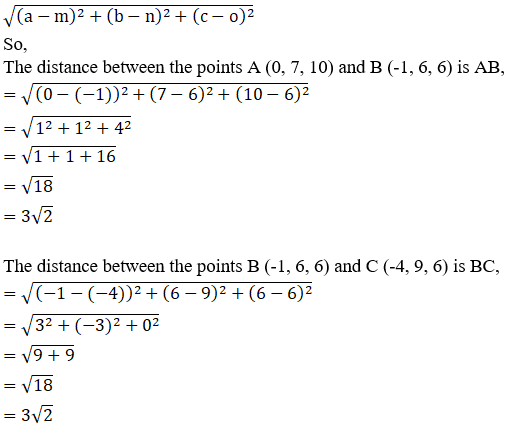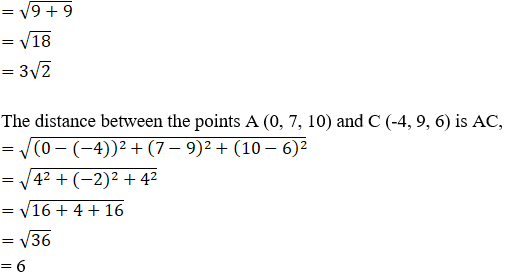Show that the points (0, 7, 10), (-1, 6, 6) and (-4, 9, 6) are the vertices of an isosceles right-angled triangle.

Asked by Sakshi | 1 year ago |  46

##### Solution :-

Given:

The points (0, 7, 10), (-1, 6, 6) and (-4, 9, 6)

Isosceles right-angled triangle is a triangle whose two sides are equal and also satisfies Pythagoras Theorem.

By using the formula,

The distance between any two points (a, b, c) and (m, n, o) is given by,Since, AB = BC

So, AB2 + BC2

= $$(3\sqrt{2})^2 + (3\sqrt{2})^2$$

= 18 + 18

= 36

= AC2

We know that, AB = BC and AB2 + BC2 = AC2

So, Δ ABC is an isosceles-right angled triangle

Hence Proved.

Answered by Aaryan | 1 year ago

### Related Questions

#### A(1, 2, 3), B(0, 4, 1), C(-1, -1, -3) are the vertices of a triangle ABC. Find the point in which the bisector of the

A(1, 2, 3), B(0, 4, 1), C(-1, -1, -3) are the vertices of a triangle ABC. Find the point in which the bisector of the angle ∠BAC meets BC.

#### The mid-points of the sides of a triangle ABC are given by (-2, 3, 5), (4, -1, 7) and (6, 5, 3). Find the coordinates

The mid-points of the sides of a triangle ABC are given by (-2, 3, 5), (4, -1, 7) and (6, 5, 3). Find the coordinates of A, B and C.

#### If the points A(3, 2, -4), B(9, 8, -10) and C(5, 4, -6) are collinear, find the ratio in which C divided AB.

If the points A(3, 2, -4), B(9, 8, -10) and C(5, 4, -6) are collinear, find the ratio in which C divided AB.